Evaluation Hardware \$353.10## Design Resources

### Design & Integration File

• Schematic
• Bill of Materials
• Gerber Files

### Evaluation Hardware

Part Numbers with "Z" indicate RoHS Compliance. Boards checked are needed to evaluate this circuit.

• EV-VSWR-SDZ (\$353.10) VSWR Demo Board

### Device Drivers

Software such as C code and/or FPGA code, used to communicate with component's digital interface.

## Features & Benefits

• Return Loss Measurement System
• 1GHz to 28GHz
• Calibration Free

## Circuit Function & Benefits

The circuit shown in Figure 1 accurately measures return loss in a wireless transmitter from 1 GHz to 28 GHz without any need for system calibration.

The design is implemented on a single circuit board using a nonreflective RF switch; a microwave RF detector; and a 12-bit, precision analog-to-digital converter (ADC). To evaluate the circuit over the widest possible frequency range, a dual-port directional coupler with SMA connectors was used instead of a narrow-band, surface-mount directional coupler.

The circuit measures return loss of up to 20 dB over an input power range of 25 dB (return losses in excess of 20 dB can be measured over a smaller input power range).

A unique feature of the circuit is that it calculates return loss using a simple ratio of the digitized voltages from the RF detector, thereby eliminating the need for system calibration.Figure 1. Voltage Standing Wave Ratio (VSWR) Evaluation Board Measurement Setup (All Connections and Decoupling Not Shown)

## Circuit Description

An RF signal between 1 GHz and 28 GHz is fed through an RF coupler (Marki Microwave C10-0226) to a matched 50 Ω load or antenna as shown in Figure 1. The forward and reverse coupled ports are connected to the HMC547, a single-pole/double-throw (SPDT) nonreflective switch. The switch input is toggled between the forward and reverse coupled ports, while terminating the opposite port in 50 Ω, so that both coupled ports always see a 50 Ω load.

The output port of the RF switch drives the ADL6010, a microwave RF detector that can operate from 500 MHz to 43.5 GHz. The output voltage level of the detector is directly proportional to the amplitude of the input signal. The ADL6010 is a linear-in-V/V detector, having a nominal slope of 2.1 V/V.

The AD7091R 12-bit ADC samples the power detector output voltage at a rate of 1 MSPS. (Lower sampling rates can also be used, resulting in lower power consumption in the ADC).

The AD7091R converts the analog voltage to a digital code. The EVAL-SDP-CB1Z (SDP-B) interface board then uses serial peripheral interface (SPI) communications to control the ADC and sends results to a PC for system evaluation and return loss calculation. The VSWR, return loss, and reflection coefficient are then calculated using the ratio between the forward and reverse coupled voltages sampled by the ADC.

### Return Loss Calculation

The following derivation shows the relationship between the ratio of forward and reverse voltages and the return loss of the system. This relationship is fundamental to the calibration-free nature of the system.

The system transfer function of the detector in its linear operating region can be expressed using the familiar straight-line equation,where:

m is the slope.
c is the intercept.

Using actual circuit parameters,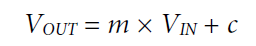As noted previously, m is nominally 2.1, but can vary with frequency and from device to device. The value of c is typically close to zero.

Rewriting Equation 1 in terms of VIN,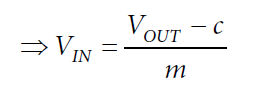Converting this equation to power,Then converting to dBm,If the ADC is included, the equation becomes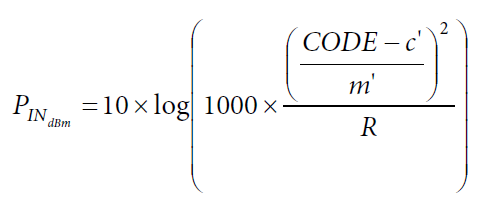where:

m’ is the slope of the detector and ADC combined signal chain.
c’ is the intercept of the detector and ADC combined signal chain.

The return loss is the difference between the forward and reverse power in dBm:Because c’ is close to zero, and because CODEF and CODER are generally much greater than c’, the formula reduces to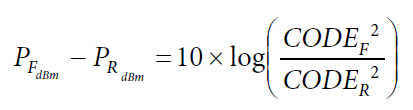The derivation in this section shows that return loss can be calculated without the need for calibration, because the formula does not include the slope (m’) or intercept (c’) of the signal chain.

### RF Switch

The HMC547 is a nonreflective SPDT RF switch with a frequency range of dc to up to 28 GHz. As shown in the Figure 2 block diagram, the switch internally terminates either input at 50 Ω, while the other input is fed to the RFC output. The switch has a fast switching time of typically 6 ns. The A and B logic inputs of this switch are controlled by negative voltage logic of −5 V high and 0 V low. A recommended control circuit is included in the HMC547 data sheet. The circuit consists of a 5.1 V Zener diode level shifter that drives 74LV04AD inverters. The inverters are powered between −5 V and 0 V, rather than 0 V and +5 V. The complete power supply circuit is shown in the detailed schematic contained in the CN-0387 Design Support Package, available at www.analog.com/CN0387-DesignSupport.

### Power Detector

The ADL6010 power detector has a linear-in-V/V characteristic, which is key to this application. To power this device, apply a +5 V dc voltage to the VPOS pin and round to the COMM pin, as shown in Figure 3.

As shown in Figure 4, the output voltage varies with frequency. This variation in transfer function vs. frequency does not degrade the performance of the circuit in any way because the return loss calculation relies on a ratiometric calculation at a specific frequency.Figure 4. Transfer Function at Frequencies from 500 MHz to 43.5 GHz

### Analog-to-Digital Converter

The AD7091R is a 12-bit successive approximation register (SAR) ADC, which has a throughput rate of up to 1 MSPS. Although a highly accurate external reference voltage can be used, it is not required in this application. In this circuit, the internal reference of 2.5 V is used, which yields an LSB size of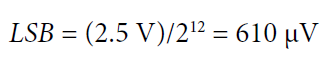Because the output voltage of the ADL6010 can reach a maximum voltage of approximately 3 V, it is necessary to attenuate this voltage using a 200 Ω/340 Ω resistor divider between the detector and the ADC, as shown in Figure 1. This divider provides a nominal attenuation of 1.6.

### Directional Coupler

A directional coupler couples a portion of the forward or reverse signals to the power detector for measurement. In general, couplers have 4 ports as shown in Figure 6.

In the Figure 6 configuration, the input signal is coupled to Port 4, and Port 3 is terminated at 50 Ω for nonreflective coupling of the signal. If Port 4 is terminated in 50 Ω instead of Port 3, the reflected signal is coupled to Port 3.

In this circuit, instead of using the 50 Ω termination connected directly to the port as shown previously, both ports are fed to the RF switch inputs. Therefore, the coupler can be regarded as being bidirectional, because the 50 Ω termination is applied by the HCM547 internally to Port 3 or Port 4, depending on the state of the switch.

The RF coupler chosen for this circuit was the Marki Microwave C10-0226 stripline coupler. This coupler has 10 dB coupling, meaning that the coupled signal is 10 dB less than the input signal. A directional coupler with SMA connectors was used in this circuit to demonstrate operation over the widest possible frequency range. A surface-mount coupler can also be used; however, such devices generally have a narrower frequency range.

### Data Analysis

The EVAL-SDP-CB1Z system demonstration platform (SDP) board is used in conjunction with evaluation software, to capture the data being sampled by the ADC.

The software calculates the return loss using Equation 8, which was derived previously. The reflection coefficient and VSWR are derived from this equation.Figure 7 shows the result display panel of the software GUI.

### Detector Sampling Strategy

To accurately measure the return loss of the system, the forward and reverse voltages must be measured with a short time delay between the forward and reverse measurements. Figure 8 shows the sampling sequence performed when sampling continuously.

When the RF switch receives the signal to toggle the switch, the switch position changes, and the forward or reverse coupled port signal is fed to the power detector. In the return loss calculation step, 500 forward samples and 500 reverse samples are averaged, and the return loss is calculated from the ratio of averaged forward and reverse voltages.

The ADC samples at a rate of 1 MSPS. Therefore, it takes 500 μs to measure 500 samples. Toggling the switch position using a general-purpose input/output (GPIO) of the SDP-B interface takes approximately 400 μs between forward and reverse cycles. The timing diagram is shown in Figure 9.The return loss, reflection coefficient, and VSWR are calculated using the resultant average of forward and reverse voltage measurements. To allow results to be clearly read before updating, 50 sample results are averaged before displaying on the GUI results panel.

Complete documentation for the EVAL-VSWR-SDZ board, including schematics, layouts, Gerber files, and bill of materials, is available for download in the CN-0387 Design Support Package at www.analog.com/CN0387-DesignSupport.

## Common Variations

As previously noted, a wideband connectorized directional coupler was used in this circuit to enable operation over the widest possible frequency range. Surface-mount directional couplers or printed circuit directional couplers can also be used; however, these devices tend to have narrower frequency ranges.

Directional couplers with higher coupling factors can also be used, which tend to have lower insertion loss. However, it is recommended that the circuit be dimensioned to maximize measurement range. For example, if the maximum system power is +35 dBm, using a 20 dB directional coupler sets the maximum power to the detector at approximately +15 dBm, which is at the upper limit of the input range.

## Circuit Evaluation & Test

### Equipment Needed

The following equipment is needed to perform the evaluations described in this circuit note:

• The EV-VSWR-SDZ evaluation board.
• A suitably rated broadband RF Coupler. For this evaluation, the Marki Microwave C10-0226 was used. However, any coupler with suitable specifications and with a 3.5 mm SMA type connector can be used.
• The EVAL-SDP-CB1Z SDP-B board.
• A signal generator (with output frequency within the range of 500 MHz to 28 GHz).
• A suitable power supply unit with 6 V dc output voltage.
• An SMA attenuator and 50 Ω termination.
• A PC running Windows® 7 connected to the SDP-B board via a USB cable (supplied with the EVAL-SDP-CB1Z).

### Setup and Test

To set up and test the VSWR measurement system, set LK6 in position B and put LK22 in place, then take the following steps:

1. Turn on all test equipment and wait until all equipment boots up.
2. Connect the input and output coupled ports of the RF coupler to the RF1 and RF2 3.5 mm SMA connections of the evaluation board, respectively, using suitably rated RF cables.
3. Connect the coupler input port to the 50 Ω output of the signal generator.
4. Connect the output of the coupler to a 50 Ω termination or a suitably rated RF attenuator termination.
5. Connect the EVAL-SDP-CB1Z SDP interface board to the EV-VSWR-SDZ evaluation board.
6. Connect the SDP interface board to a PC via the USB cable provided.
7. Connect the power supply from the dc supply to the power and ground of the banana sockets of the evaluation board.
8. Download and install the CN-0387 Evaluation Software onto the PC that is connected to the SDP-B control board.
9. After the software is installed properly, run the executable.
10. Set the output frequency of the signal generator to 2 GHz and set the power level to 15 dBm output. Then turn on the output of the signal generator.
11. In the software display, select Continuous, and then click Capture. The software continuously repeats measurements; when each measurement is gathered, the GUI display updates with the value of the return loss and the corresponding values of VSWR and reflection coefficient

### Functional Block Diagram of Test Setup

Figure 10 shows the functional block diagram of the test setup.

### Test Results

Measurements were gathered manually by adjusting the RF input power levels. Return loss in different output configurations was measured over the power range of the ADL6010.

RF simulations were also run using the Keysight Advanced Design System (ADS). This software is an electronic design simulation tool for RF and microwave applications. Simulations were performed to verify that RF input trace insertion loss and reflection were within certain limits and to simulate the performance of the directional coupler.

The Marki Microwave C10-0226 directional coupler was provided with a simulation model file, an .s4p file. This file contains information that describes the S-parameters of the coupler and can be readily used in ADS within a simulation. Simulations were performed over a frequency range from dc up to the upper frequency limit of the coupler of 26.5 GHz, and the input power was set to 0 dBm for each simulation.

### Return Loss Measurement

While testing the EV-VSWR-SDZ evaluation board, a 9 dB attenuator termination was connected to the coupler output to verify that the expected attenuation levels were measured at a chosen frequency as compared to the simulated results.

As shown in Figure 11, within the input power range of 0 dBm to 25 dBm, the return loss measured remains nearly constant at 20 dB. This value is the summation of the forward and reverse attenuations as previously outlined (9 dB + 9 dB), and the forward insertion loss of the coupler at 2 GHz using the Marki Microwave C10-0226. After the input power at the forward coupled port reaches approximately 27 dBm, the return loss reduces significantly. This reduction occurs because the coupled power at the forward coupled port is close to +15 dBm (with 10 dB coupling), which is the upper power limit of the ADL6010. As input power is reduced, the measured return loss begins to reduce due to the reflected power at the reverse coupled port dropping below the lower limit of −30 dBm. Figure 11 illustrates the power range over which the ADL6010 can operate to measure a return loss of 20 dB.

### Power Detector Measured Power

Figure 12 shows how the measured power changes with input power. A calibration routine was performed at 2 GHz to obtain accurate power measurement at the forward coupled port, at each power level. As the input power rises, the measured power reaches the detector limit of 15 dBm. Similarly, as input power is reduced, the lower limit of the detector is reached and measurement accuracy reduces.

### Open Circuit Configuration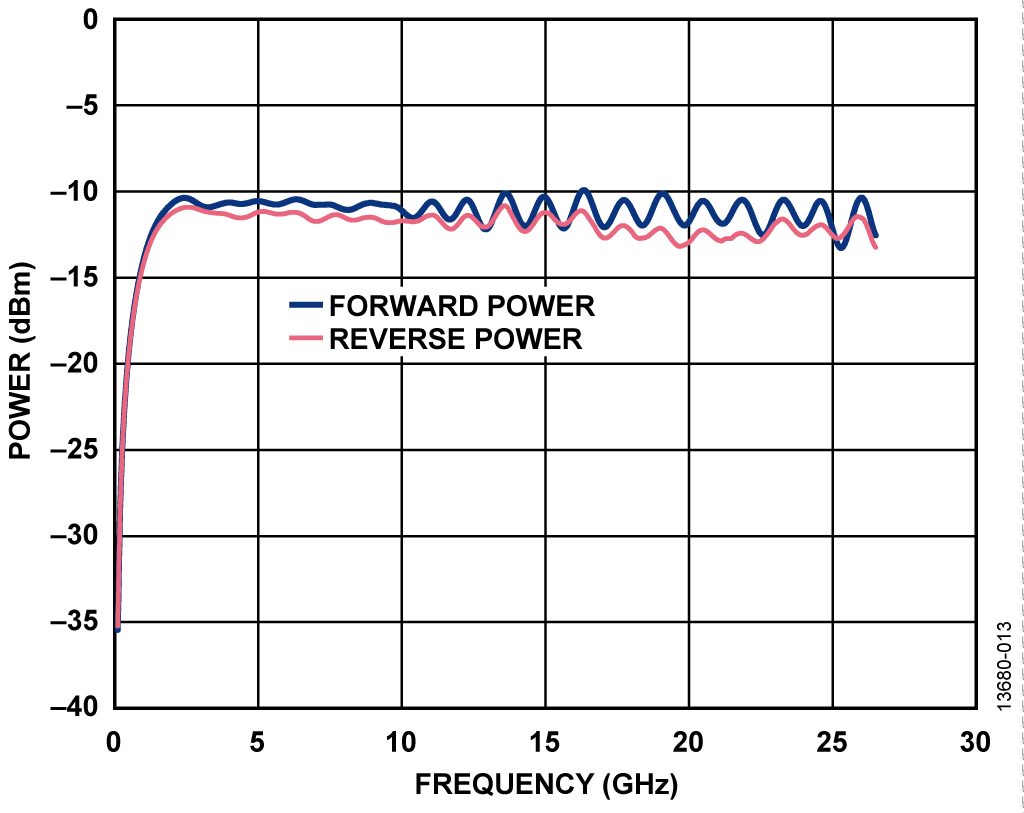Figure 13. Coupler Forward and Reverse Power vs. Frequency—Open Circuit

Figure 13 shows that the return loss is close to 0 dB as forward and reverse power levels are close in value, compared to the previous 50 Ω termination case.

This open-circuit simulation shows how the majority of the signal is reflected back through the coupler in the opposite direction to the forward wave. The difference in amplitude between the forward and reflected waves is a result of the insertion loss of the coupler across the frequency range. This graph highlights that exact return loss and VSWR measurements vary with the coupler used, due to non-ideal coupler impedance matching across frequency.

Additionally, a threshold return loss can be set to indicate whether there is a mismatch occurring in the system, which is much greater than the coupler return loss.

### 50 Ω Output Termination Circuit ConfigurationFigure 14. Coupler Forward and Reverse Power vs. Frequency—50 Ω Termination

Figure 14 shows the Marki Microwave C10-0226 RF coupler forward insertion loss and reflected signal for a 50 Ω terminated output with a 0 dBm power level applied to the forward port of the coupler. The coupler is specified to operate from 2 GHz to 26.5 GHz. Therefore, the coupler begins to operate as expected beyond 2 GHz in Figure 14. The simulation uses an ideally matched transmission line of 50 Ω output impedance on the coupler. The return loss at any frequency is the difference between the forward and reverse power. The graph shows that return loss varies with frequency. However, within this frequency range, the return loss is close to 20 dB, which is acceptable because return loss values greater than 20 dB are taken to be negligible.

### 3 dB Output Attenuator Circuit Configuration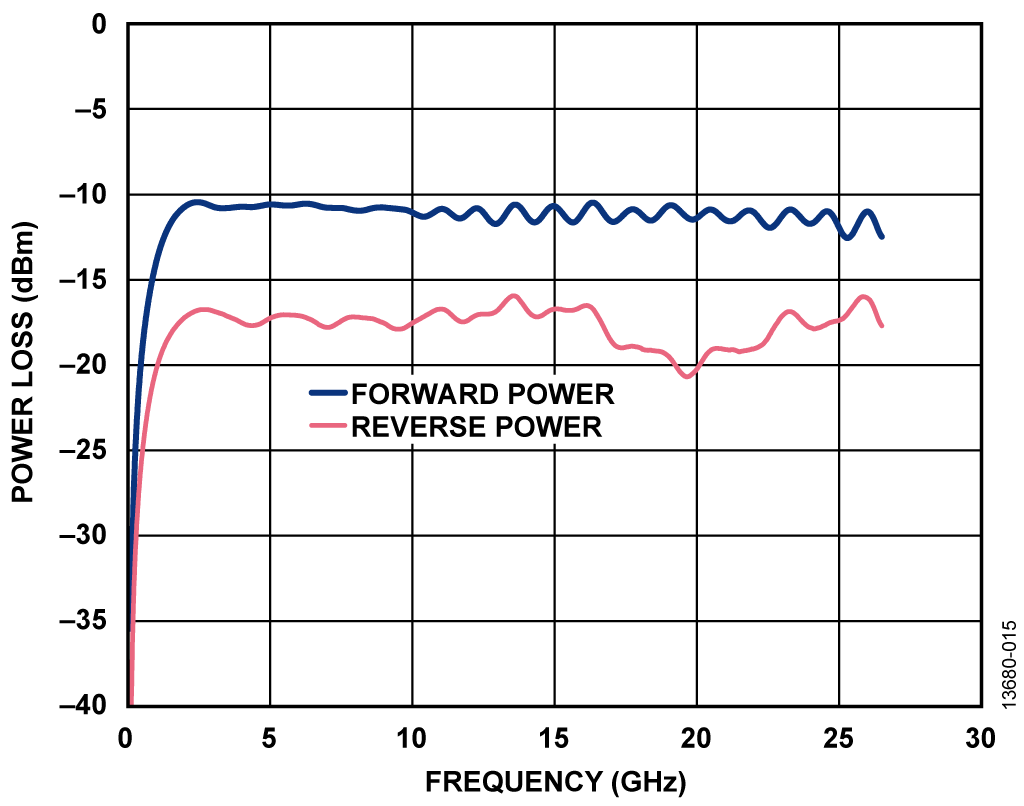Figure 15. Coupler Forward and Reverse Power vs. Frequency—3 dB Attenuator

In Figure 15, the return loss is relatively stable at 6 dB until up to approximately 15 GHz, after which the loss of the coupler increases and the attenuation increases.

The 6 dB attenuation is due to the 3 dB coupler attenuating both the incident signal and reflected signal by 3 dB. As shown in Figure 16, the signal first attenuates by 3 dB in the forward direction before being reflected and attenuated by a further 3 dB. The measured reflected signal is the result of the accumulated forward and reverse attenuations.

### Calibration Structure Trace Insertion Loss

The RF trace insertion loss was simulated using the layout file of the EV-VSWR-SDZ evaluation board. This layout file was imported into the ADS tool to run a simulation, to determine the losses of the RF traces on the board.

The calibration structure shown in Figure 17 on the board section was used in the simulation. This structure was included in the board design to measure the losses from the SMA connector to the switch (Distance A). As shown, the length of the calibration structure is exactly twice the distance from RF1/RF2 to the HMC547 switch (U10). To measure these RF trace losses at a particular frequency, the signal is applied to one of the calibration structure connectors and is then measured on the opposite connector.

The ADS simulation tool was run on this structure from dc to 26.5 GHz to simulate the insertion loss of the RF traces.

As shown in Figure 18, the insertion loss of the calibration structure reaches a maximum attenuation of approximately −0.4 dB at a frequency of 26.5 GHz.

This insertion loss is considered to be within the acceptable limits for the evaluation board. If this insertion loss is significant, it limits the range of measurement.

A photograph of the EV-VSWR-SDZ board connected to the EVAL-SDP-CB1Z board is shown in Figure 19.

An electrically equivalent replacement part for the HMC547LC3 will be available in June 2016.

## Evaluation Boards

Pricing displayed is based on 1-piece.

Up to two boards can be purchased through Analog.com. To order more than two, please purchase through one of our listed distributors.

Pricing displayed is based on 1-piece. The USA list pricing shown is for budgetary use only, shown in United States dollars (FOB USA per unit), and is subject to change. International prices may vary due to local duties, taxes, fees and exchange rates.